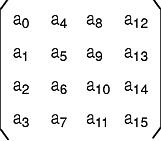[ Previous | Next | Contents | Glossary | Home | Search ]
OpenGL 1.1 for AIX: Reference Manual

## glMultMatrix Subroutine

### Purpose

Multiplies the current matrix by an arbitrary matrix.

### Library

OpenGL C bindings library: libGL.a

### C Syntax

`void glMultMatrixd(const GLdouble *Matrix)`
`void glMultMatrixf(const GLfloat *Matrix)`

### Parameters

 Matrix Specifies a pointer to 4 x 4 matrix stored in column-major order as 16 consecutive values.

### Description

The glMultMatrix subroutine multiplies the current matrix with the one specified in the Matrix parameter. For example, if M is the current matrix and T is the matrix passed to glMultMatrix, M is replaced with MT.

The current matrix is the projection matrix, model view matrix, or texture matrix, determined by the current matrix mode. (See the glMatrixMode subroutine for information on specifying the current matrix.)

The Matrix parameter points to a 4 x 4 matrix of single- or double-precision floating-point values stored in column-major order. That is, the matrix is stored as in the following figure:### Errors

 GL_INVALID_OPERATION The glMultMatrix subroutine is called between a call to glBegin and the corresponding call to glEnd.

### Associated Gets

Associated gets for the glMultMatrix subroutine are as follows. (See the glGet subroutine for more information.)

glGet with argument GL_MATRIX_MODE

glGet with argument GL_MODELVIEW_MATRIX

glGet with argument GL_PROJECTION_MATRIX

glGet with argument GL_TEXTURE_MATRIX.

### Files

 /usr/include/GL/gl.h Contains C language constants, variable type definitions, and ANSI function prototypes for OpenGL.

### Related Information

The glBegin or glEnd subroutine, glLoadIdentity subroutine, glLoadMatrix subroutine, glMatrixMode subroutine, glPushMatrix subroutine.

[ Previous | Next | Contents | Glossary | Home | Search ]